# Chapter 39 Mixed models

Motivating scenario: We want to estimate account forthe variability among groups when making inference.

## 39.1 Review

### 39.1.1 Linear models

Remember, linear models predict Y as a deviation from some constant, a, as a function of a bunch of stuff.

$\widehat{Y_i} = a + b_1 \times y_{1,i} + b_2 \times y_{2,i} + \dots$ Among the major assumptions of linear models is the independence of residuals, conditional on predictors. But in the real world, this assumption is often violated.

## 39.2 Multilevel (aka heirarchical) models

Of course, we can overcome this assumption of independence by modelling the dependence structure of the data. For example, if results of student’s tests are nondependent because they are in a class in a school we can put school and class into our model.

When we aren’t particularly interested in school or class themselves, these are called nuisance parameters.

When school (or class, or whatever “cluster” we’re concerned with) is chosen at random we can model it as a random effect. If not, we can use various other models, including generalized least squares (GLS), which we are not covering right now, but which have similar motivations.

## 39.3 Mixed effect models

How do we test for some fixed effect, like if type of math instruction increases math scores, in the presence of this nonindependence (like students in the same class learn from the same teacher etc…)?

In a mixed effect model, we model both fixed and random effects!!!!

### 39.3.1 Random Intercepts

With a “random intercept” each cluster gets its own intercept, which it drawn from some distribution. This on its own is much like the example in Chapter 38.

If we assume a random intercept only, we assume that each cluster draws an intercept from some distribution, but that clusters have the same slope (save what is specified in the fixed effect).

### 39.3.2 Random Slopes

With a “random slope” each cluster gets its own slope, which it drawn from some distribution.

If we assume a random slope only, we assume that each cluster draws a slope from some distribution, but that cluster have the same intercepts (save what is specified in the fixed effect).

### 39.3.3 Random Slopes and Random Intercepts

We can, in theory model both random slopes and random intercepts. But sometimes these are hard to estimate together, so be careful.

### 39.3.4 Visualizing Random Intercepts and Random Slopes

I find it pretty hard to explain random slopes and intercepts in words, but that pictures really help!

Read and scroll through the example below (from http://mfviz.com/hierarchical-models/), as it is the best description I’ve come across.

## 39.4 Mixed model: Random intercept example

Selenium, is a bi-product of burning coal. Could Selenium bioaccumulate in fish, suchb that fish from lakes with more selenium have more selenium in them?

Lets look at data (download here) from 98 fish from nine lakes, with 1 to 34 fish per lake.

### 39.4.1 Ignoring nonindependence is inapprorpiate

What if we just ignored lake and assumed each fish was independent???

selenium     <- read_csv("https://drive.google.com/uc?export=download&id=1UAkdZYLJPWRuFrq9Dc4StxfHCGW_43Ye")
selenium_lm1 <- lm(Log_fish_Se ~  Log_Water_Se, data = selenium)

summary(selenium_lm1)
##
## Call:
## lm(formula = Log_fish_Se ~ Log_Water_Se, data = selenium)
##
## Residuals:
##     Min      1Q  Median      3Q     Max
## -0.7569 -0.3108 -0.1789  0.4301  0.8180
##
## Coefficients:
##              Estimate Std. Error t value Pr(>|t|)
## (Intercept)   1.16351    0.06387  18.216  < 2e-16 ***
## Log_Water_Se  0.26483    0.05858   4.521 2.08e-05 ***
## ---
## Signif. codes:  0 '***' 0.001 '**' 0.01 '*' 0.05 '.' 0.1 ' ' 1
##
## Residual standard error: 0.4305 on 81 degrees of freedom
## Multiple R-squared:  0.2015, Adjusted R-squared:  0.1916
## F-statistic: 20.44 on 1 and 81 DF,  p-value: 2.081e-05
anova(selenium_lm1)
## Analysis of Variance Table
##
## Response: Log_fish_Se
##              Df Sum Sq Mean Sq F value    Pr(>F)
## Log_Water_Se  1  3.787  3.7870  20.436 2.081e-05 ***
## Residuals    81 15.010  0.1853
## ---
## Signif. codes:  0 '***' 0.001 '**' 0.01 '*' 0.05 '.' 0.1 ' ' 1
ggplot(selenium, aes(x = Log_Water_Se, y = Log_fish_Se, label = Lake)) +
geom_point(aes(color = Lake), alpha = .5, size = 3) +
geom_smooth(method = "lm")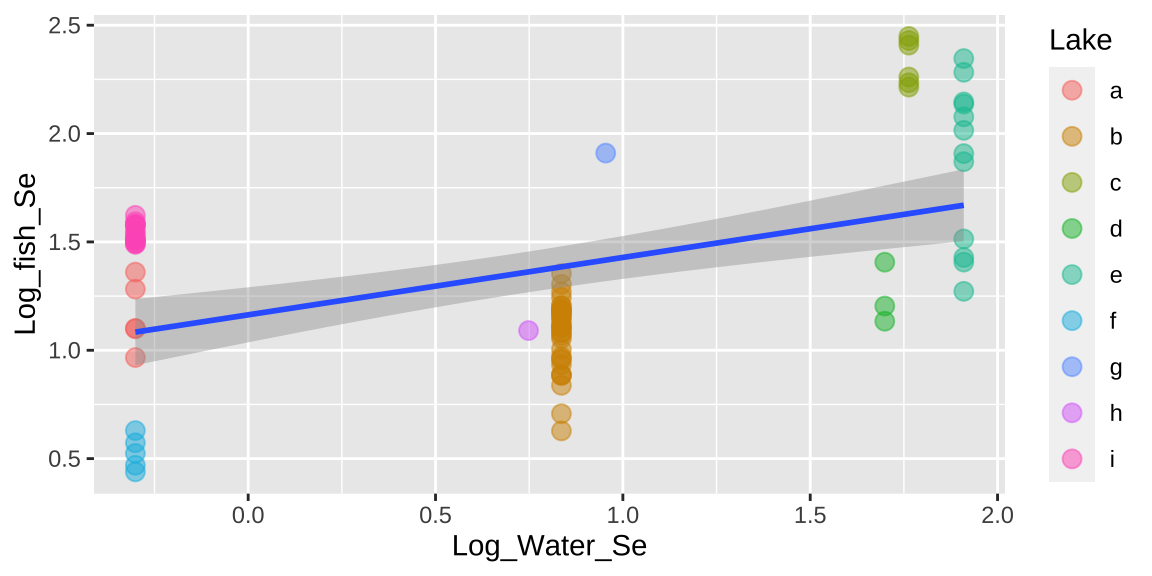That would be a big mistake – as each fish is not independent. There could be something else about the lake associated with levels of Selenium in fish that is not in our model. This seems likely, as we see below, that residuals differ substantially by lake.

bind_cols( selenium ,
augment(selenium_lm1)  %>%    dplyr::select(.resid))%>%
ggplot(aes(x = Lake, y = .resid, color = Lake)) +
geom_jitter(width = .2, size = 3, alpha =.6, show.legend = FALSE)+
geom_hline(yintercept = 0, color = "red")+
stat_summary(data = . %>% group_by(Lake) %>% mutate(n = n()) %>% dplyr::filter(n>1), color = "black")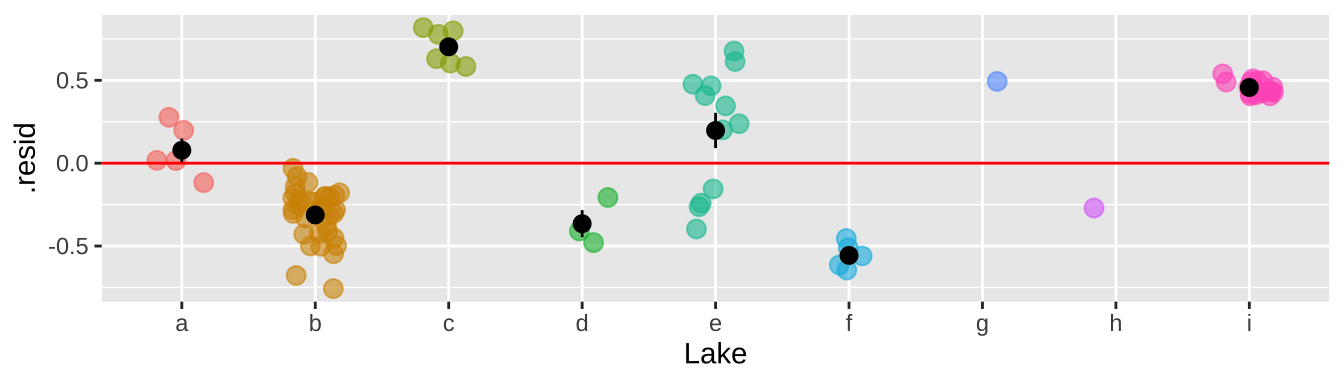So why is this so bad? It’s because we keep pretending each fish is independent when it is not. So, our certainty is WAY bigger than it deserves to be. This means that our p-value is WAY smaller than it deserves to be.

### 39.4.2 We can’t model lake as a fixed effect

And even if we could it would be wrong.

Putting lake into our model as a fixed effect would essentially take the average of Fish Selenium content for each lake. Since each lake has only one Selenium content, we then have nothing to model.

lm(Log_fish_Se ~  Lake + Log_Water_Se, data = selenium) 
##
## Call:
## lm(formula = Log_fish_Se ~ Lake + Log_Water_Se, data = selenium)
##
## Coefficients:
##  (Intercept)         Lakeb         Lakec         Laked         Lakee         Lakef         Lakeg
##      1.16191      -0.08949       1.17069       0.08629       0.70488      -0.63493       0.74802
##        Lakeh         Lakei  Log_Water_Se
##     -0.07097       0.37860            NA

But even when this is not the case, this is not the appropriate modelling strategy.

### 39.4.3 Taking averages is a less wrong thing

What if we just took the average selenium content over all fish for every lake, and then did our stats?

selenium_avg <- selenium %>%
group_by(Lake, Log_Water_Se) %>%
summarise(mean_log_fish_Se = mean(Log_fish_Se), n = n())

selenium_lm2 <- lm(mean_log_fish_Se ~  Log_Water_Se, data = selenium_avg)
summary(selenium_lm2)
##
## Call:
## lm(formula = mean_log_fish_Se ~ Log_Water_Se, data = selenium_avg)
##
## Residuals:
##      Min       1Q   Median       3Q      Max
## -0.50657 -0.36536  0.03456  0.42871  0.55425
##
## Coefficients:
##              Estimate Std. Error t value Pr(>|t|)
## (Intercept)    1.1307     0.2094   5.399  0.00101 **
## Log_Water_Se   0.3673     0.1807   2.032  0.08161 .
## ---
## Signif. codes:  0 '***' 0.001 '**' 0.01 '*' 0.05 '.' 0.1 ' ' 1
##
## Residual standard error: 0.4653 on 7 degrees of freedom
## Multiple R-squared:  0.3711, Adjusted R-squared:  0.2813
## F-statistic: 4.131 on 1 and 7 DF,  p-value: 0.08161
anova(selenium_lm2)
## Analysis of Variance Table
##
## Response: mean_log_fish_Se
##              Df  Sum Sq Mean Sq F value  Pr(>F)
## Log_Water_Se  1 0.89428 0.89428   4.131 0.08161 .
## Residuals     7 1.51536 0.21648
## ---
## Signif. codes:  0 '***' 0.001 '**' 0.01 '*' 0.05 '.' 0.1 ' ' 1
ggplot(selenium_avg, aes(x = Log_Water_Se, y = mean_log_fish_Se, label = Lake)) +
geom_point(aes(size = n)) +
geom_text_repel(aes(color = Lake), show.legend = FALSE) +
geom_smooth(method = "lm",se = FALSE)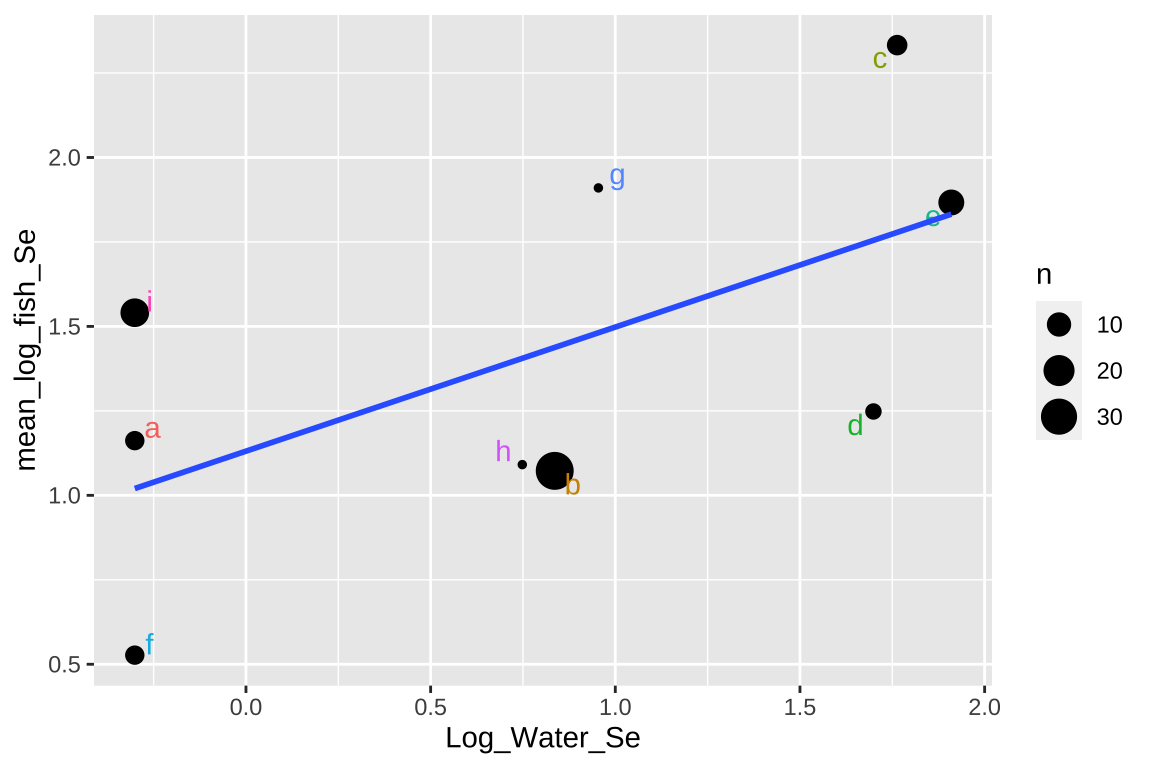This approach isn’t too bad, but there are some shortcomings –

1. It takes all means as equally informative, even though some come from large and others from large samples.
2. It ignores variability within each lake,
3. It surrenders so much of our hard-won data.

### 39.4.4 Accounting for Lake as a Random Effect is our best option

Rather, we can model Lake as a random effect – this allows us to model the non-independence of observations within a Lake, while using all of the data.

selenium_lme <- lmer(Log_fish_Se ~   (1|Lake) + Log_Water_Se , data = selenium)
summary(selenium_lme)
## Linear mixed model fit by REML. t-tests use Satterthwaite's method ['lmerModLmerTest']
## Formula: Log_fish_Se ~ (1 | Lake) + Log_Water_Se
##    Data: selenium
##
## REML criterion at convergence: -8.8
##
## Scaled residuals:
##      Min       1Q   Median       3Q      Max
## -3.15542 -0.37400  0.04239  0.54953  2.54730
##
## Random effects:
##  Groups   Name        Variance Std.Dev.
##  Lake     (Intercept) 0.20747  0.4555
##  Residual             0.03548  0.1884
## Number of obs: 83, groups:  Lake, 9
##
## Fixed effects:
##              Estimate Std. Error     df t value Pr(>|t|)
## (Intercept)    1.1322     0.2089 6.8420   5.419  0.00107 **
## Log_Water_Se   0.3659     0.1794 6.7266   2.039  0.08249 .
## ---
## Signif. codes:  0 '***' 0.001 '**' 0.01 '*' 0.05 '.' 0.1 ' ' 1
##
## Correlation of Fixed Effects:
##             (Intr)
## Log_Water_S -0.666

Thus we fail to reject the null, and conclude that there is not sufficient evidence to support the idea that more selenium in lakes is associated with more selenium in fish.

#### 39.4.4.1 Extracting estimates and uncertainty

Alhough we do have model estimates, degrees of freedom and standard deviations above, if ca still be hard to consider and commuincate uncertainty. The ggpredict function in the ggeffects package can help!

library(ggeffects)
gg_color_hue <- function(n) {hues = seq(15, 375, length = n + 1); hcl(h = hues, l = 65, c = 100)[1:n]}

selenium_predictions <- ggpredict(selenium_lme, terms = "Log_Water_Se")
selenium_predictions
## # Predicted values of Log_fish_Se
## # x = Log_Water_Se
##
##     x | Predicted |       95% CI
## --------------------------------
## -0.30 |      1.02 | [0.54, 1.51]
##  0.75 |      1.41 | [1.10, 1.71]
##  0.84 |      1.44 | [1.13, 1.74]
##  0.95 |      1.48 | [1.17, 1.79]
##  1.70 |      1.75 | [1.31, 2.20]
##  1.76 |      1.78 | [1.31, 2.24]
##  1.91 |      1.83 | [1.33, 2.33]
##
## Adjusted for:
## * Lake = 0 (population-level)
plot(selenium_predictions)+
geom_point(data = selenium, aes(x = Log_Water_Se , y= Log_fish_Se, color = Lake), alpha = .4, size = 3)+
scale_color_manual(values = gg_color_hue(9))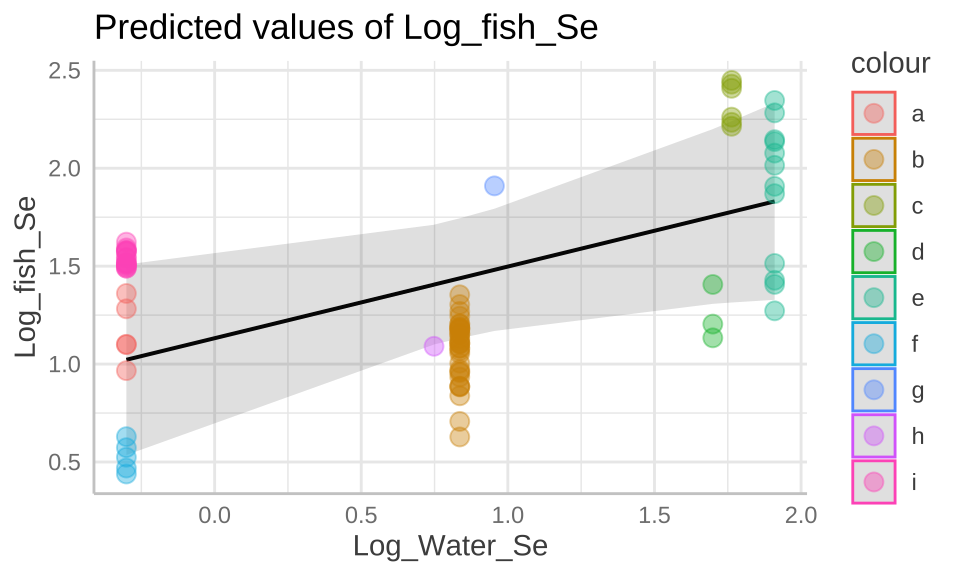#### 39.4.4.2 Evaluating assumptions

We can now see that by modeling Lake as a random effect, the residuals are now independent.

bind_cols( selenium ,
broom.mixed::augment(selenium_lme)  %>%    dplyr::select(.resid))%>%
ggplot(aes(x = Lake, y = .resid, color = Lake)) +
geom_jitter(width = .2, size = 3, alpha =.6, show.legend = FALSE)+
geom_hline(yintercept = 0, color = "red")+
stat_summary(data = . %>% group_by(Lake) %>% mutate(n = n()) %>% dplyr::filter(n>1),color = "black")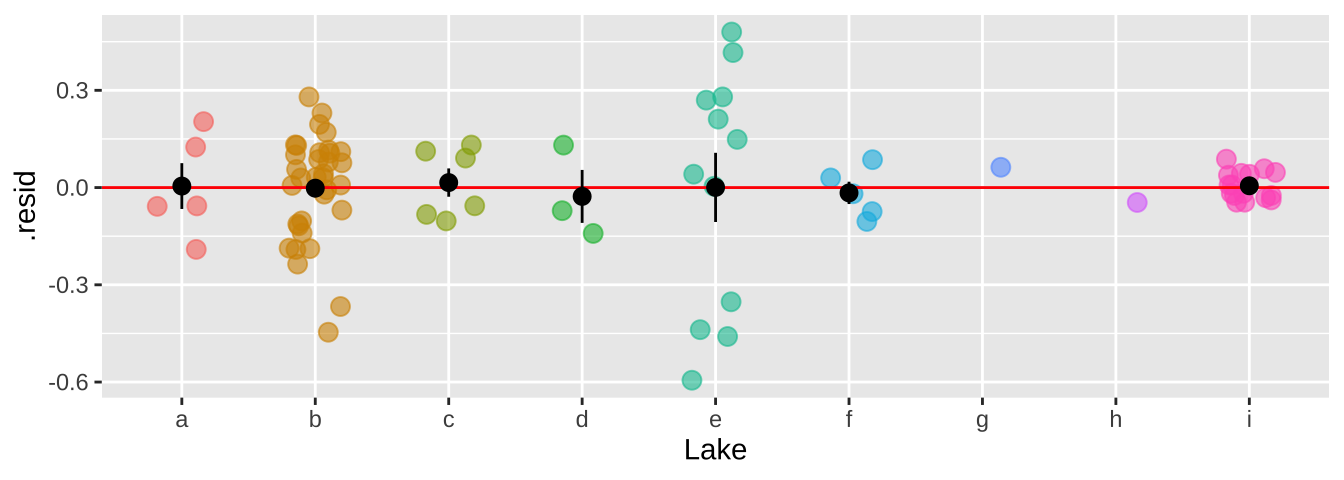Major assumptions of linear mixed effect models are

• Data are collected without bias
• Clausters are random
• Data cna be modelled as a linear function of predictors
• Variance of the reiduals is indpednet of predicted values,
• Residuals are normally distributed,

Let’s look into the last two for these data. Because we hate autoplot, and it can’t handle random effects outplut, let’s make out own diangnostic plots.

selenium_qq <- broom.mixed::augment(selenium_lme) %>%
ggplot(aes(sample = .resid))+
geom_qq()+
geom_qq_line()

selenium_std_resid  <- broom.mixed::augment(selenium_lme) %>%
mutate(sqrt_abs_std_resid = sqrt(abs(.resid / sd(.resid)) )) %>%
ggplot(aes(x = .fitted, y = sqrt_abs_std_resid)) +
geom_point()

plot_grid(selenium_qq, selenium_std_resid, ncol = 2)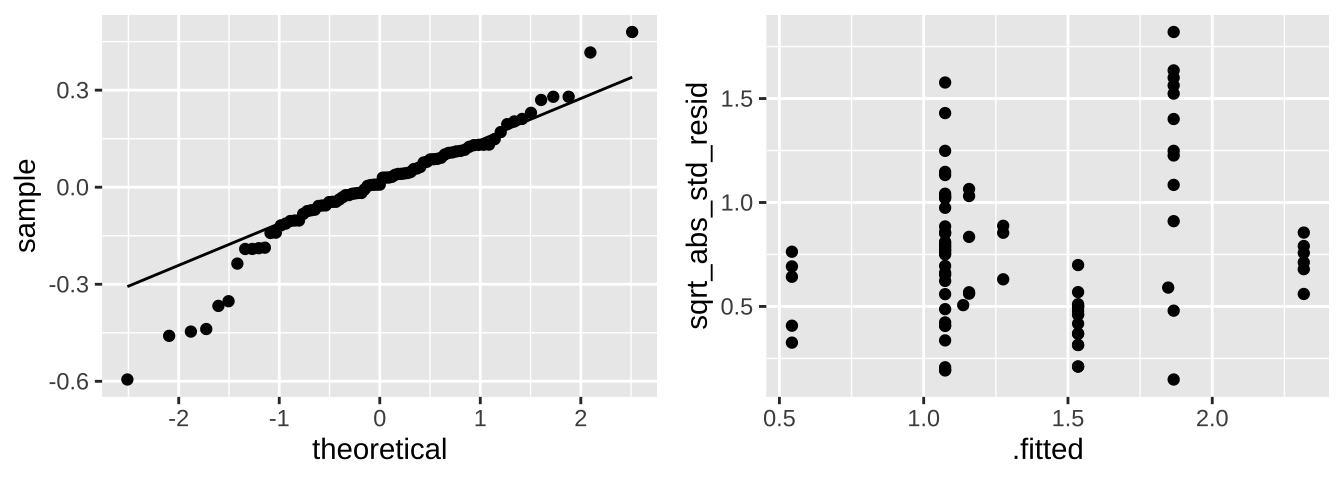These don’t look great tbh. We would need to think harder if we where doing a legitimate analysis.

## 39.5 Example II: Loblolly Pine Growth

Let’s try to model the growth rate of loblolly pines!

First I looked at the data (a), and thought a square root transfrom would be a better linear model (b), expecially if I began my model after threee years of growth (c).

Loblolly <- mutate(Loblolly, Seed = as.character(Seed))
a <- ggplot(Loblolly, aes(x = age, y = height, group = Seed, color = Seed))+ geom_line()
b <- ggplot(Loblolly, aes(x = sqrt(age), y = height, group = Seed, color = Seed))+ geom_line()
c <- ggplot(Loblolly %>% filter(age > 3), aes(x = sqrt(age), y = height, group = Seed, color = Seed))+ geom_line()
top  <- plot_grid(a+theme(legend.position = "none"),
b+theme(legend.position = "none"),
c+theme(legend.position = "none") ,
labels = c("a","b","c"), ncol = 3)
legend<- get_legend(a+ guides(color = guide_legend(nrow=2)) + theme(legend.position = "bottom", legend.direction = "horizontal") )
plot_grid(top, legend, ncol =1, rel_heights = c(8,2))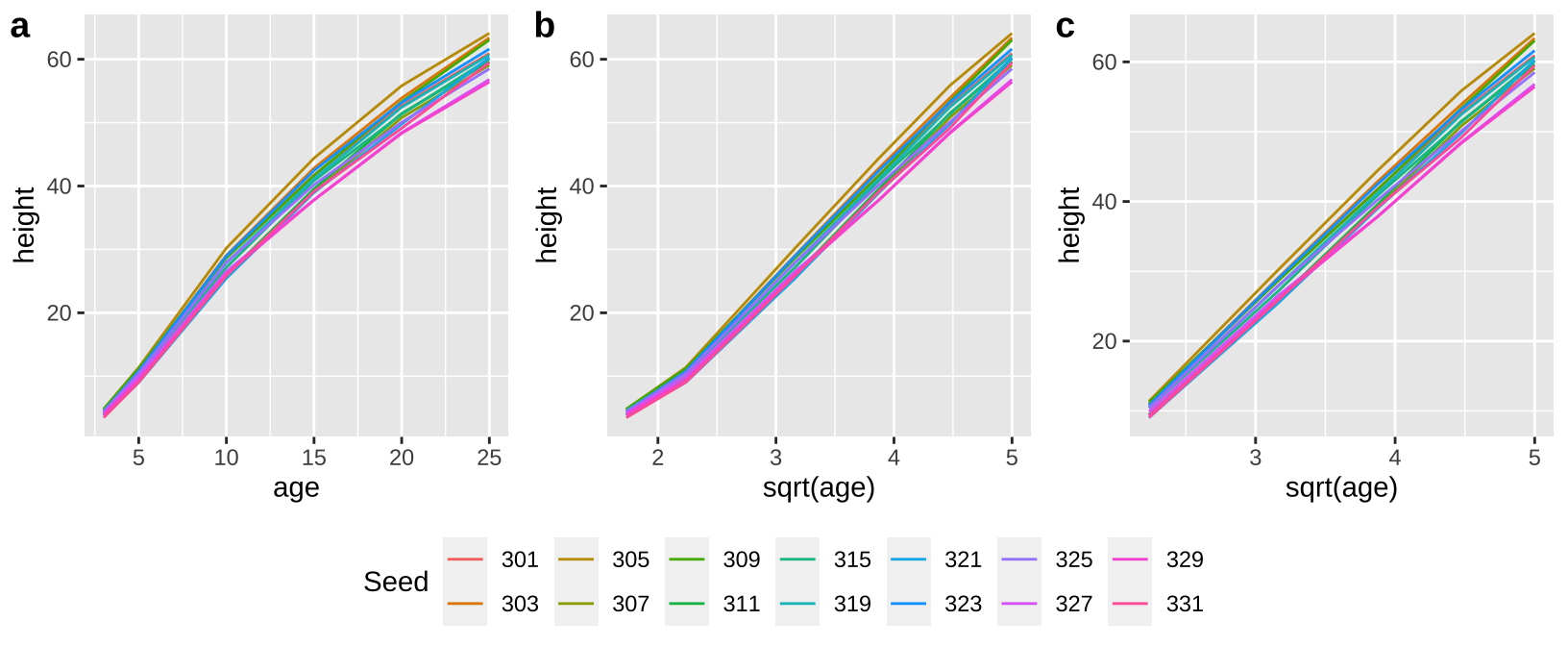### 39.5.1 Random Slopes

loblolly_rand_slope <- lmer(height ~ age + (0+age|Seed), data = filter(Loblolly, age>3))
summary(loblolly_rand_slope)
## Linear mixed model fit by REML. t-tests use Satterthwaite's method ['lmerModLmerTest']
## Formula: height ~ age + (0 + age | Seed)
##    Data: filter(Loblolly, age > 3)
##
## REML criterion at convergence: 348.6
##
## Scaled residuals:
##     Min      1Q  Median      3Q     Max
## -1.6115 -0.9585  0.1945  0.7035  1.8126
##
## Random effects:
##  Groups   Name Variance Std.Dev.
##  Seed     age  0.005779 0.07602
##  Residual      7.116593 2.66769
## Number of obs: 70, groups:  Seed, 14
##
## Fixed effects:
##             Estimate Std. Error       df t value Pr(>|t|)
## (Intercept)  0.73121    0.74777 55.00001   0.978    0.332
## age          2.48390    0.04946 61.41943  50.222   <2e-16 ***
## ---
## Signif. codes:  0 '***' 0.001 '**' 0.01 '*' 0.05 '.' 0.1 ' ' 1
##
## Correlation of Fixed Effects:
##     (Intr)
## age -0.825
broom.mixed::augment(loblolly_rand_slope) %>% mutate(Seed = as.character(Seed))  %>%
ggplot(aes(x = age, y = .fitted, color = Seed)) +
geom_line() + theme(legend.position = "none")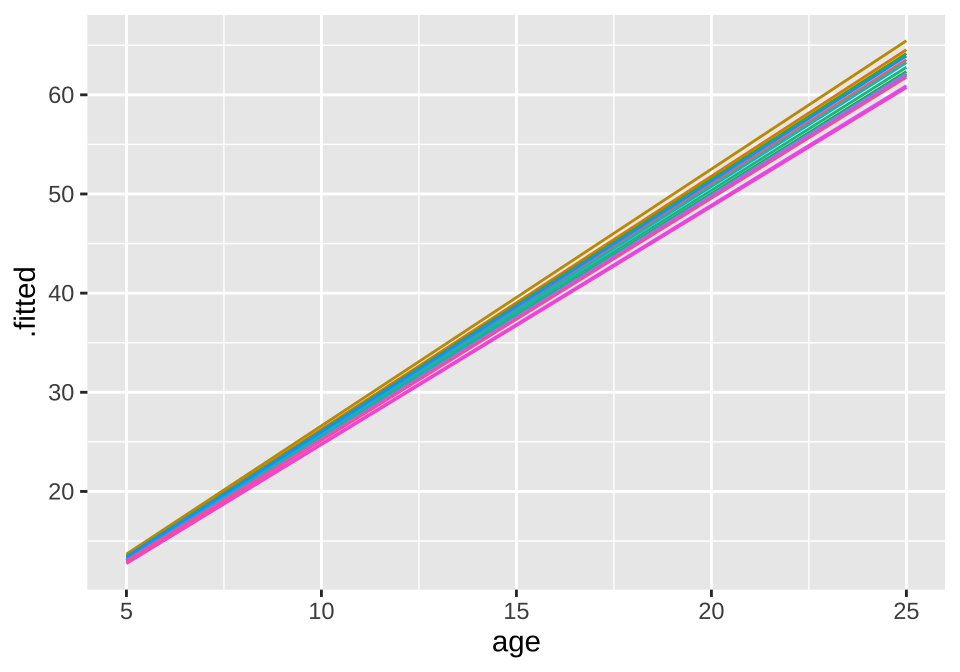### 39.5.2 Random Intercepts

loblolly_rand_intercept <- lmer(height ~ age + (1|Seed), data = filter(Loblolly, age>3))
summary(loblolly_rand_intercept)
## Linear mixed model fit by REML. t-tests use Satterthwaite's method ['lmerModLmerTest']
## Formula: height ~ age + (1 | Seed)
##    Data: filter(Loblolly, age > 3)
##
## REML criterion at convergence: 349.6
##
## Scaled residuals:
##     Min      1Q  Median      3Q     Max
## -1.9391 -0.9836  0.2641  0.7693  1.8060
##
## Random effects:
##  Groups   Name        Variance Std.Dev.
##  Seed     (Intercept) 1.318    1.148
##  Residual             7.376    2.716
## Number of obs: 70, groups:  Seed, 14
##
## Fixed effects:
##             Estimate Std. Error       df t value Pr(>|t|)
## (Intercept)  0.73121    0.82078 63.47415   0.891    0.376
## age          2.48390    0.04591 55.00000  54.109   <2e-16 ***
## ---
## Signif. codes:  0 '***' 0.001 '**' 0.01 '*' 0.05 '.' 0.1 ' ' 1
##
## Correlation of Fixed Effects:
##     (Intr)
## age -0.839
broom.mixed::augment(loblolly_rand_intercept) %>% mutate(Seed = as.character(Seed))  %>%
ggplot(aes(x = age, y = .fitted, color = Seed)) +
geom_line() + theme(legend.position = "none")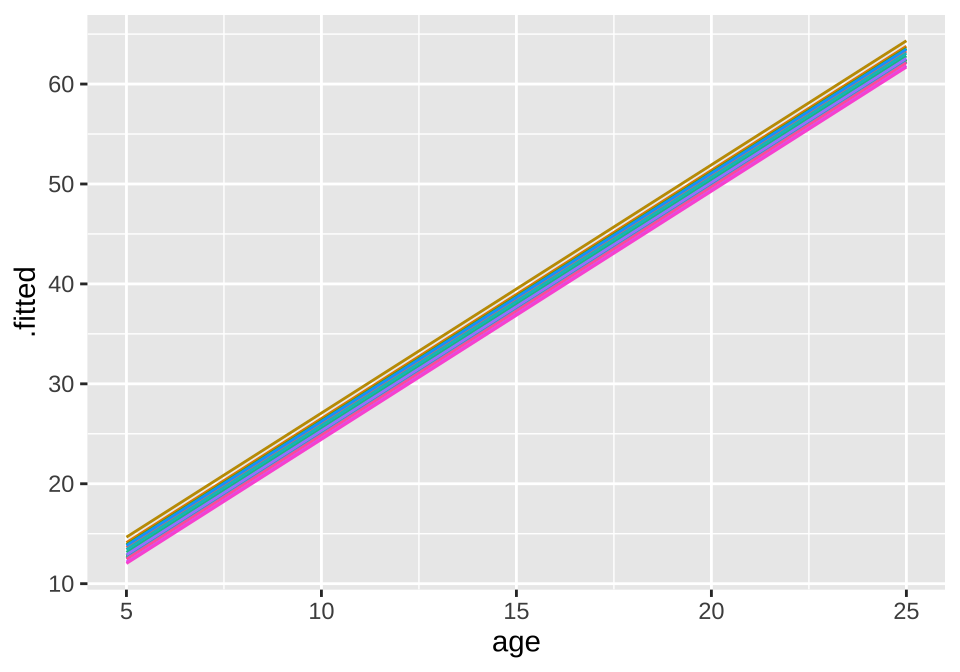## 39.7 Quiz

Amrhein, V, S Greenland, and B McShane. 2019. “Scientists Rise up Against Statistical Significance.” Nature 567 (7748): 305.
Beall, Cynthia M. 2006. Andean, Tibetan, and Ethiopian patterns of adaptation to high-altitude hypoxia.” Integrative and Comparative Biology 46 (1): 18–24. https://doi.org/10.1093/icb/icj004.
Bergstrom, Carl T, and Jevin D West. 2020. Calling Bullshit: The Art of Skepticism in a Data-Driven World. Random House. https://www.callingbullshit.org/.
Brandvain, Yaniv, and Graham Coop. 2015. “Sperm Should Evolve to Make Female Meiosis Fair.” Evolution 69 (4): 1004–14. https://doi.org/https://doi.org/10.1111/evo.12621.
Broman, Karl W., and Kara H. Woo. 2018. “Data Organization in Spreadsheets.” The American Statistician 72 (1): 2–10. https://doi.org/10.1080/00031305.2017.1375989.
Chang, Winston. 2020. “R Graphics Cookbook: Practical Recipes for Visualizing Data.” https://r-graphics.org/.
Chen, I-Ching, Jane K. Hill, Ralf Ohlemüller, David B. Roy, and Chris D. Thomas. 2011. “Rapid Range Shifts of Species Associated with High Levels of Climate Warming.” Science 333 (6045): 1024–26. https://doi.org/10.1126/science.1206432.
Clopper, C. J., and E. S. Pearson. 1934. the use of confidence or fiducial limits illustrated in the case of the binomial.” Biometrika 26 (4): 404–13. https://doi.org/10.1093/biomet/26.4.404.
Cochran, James J. 2019. “What Is the Bootstrap?” Significance 16 (1): 8–9. https://doi.org/https://doi.org/10.1111/j.1740-9713.2019.01225.x.
Collaboration, Open Science. 2015. “Estimating the Reproducibility of Psychological Science.” Science 349 (6251). https://doi.org/10.1126/science.aac4716.
Cratsley, Christopher K, and Sara M Lewis. 2003. “Female Preference for Male Courtship Flashes in Photinus Ignitus Fireflies.” Behavioral Ecology 14 (1): 135–40.
D’Hont, Angélique, France Denoeud, Jean-Marc Aury, Franc-Christophe Baurens, Françoise Carreel, Olivier Garsmeur, Benjamin Noel, et al. 2012. “The Banana (Musa Acuminata) Genome and the Evolution of Monocotyledonous Plants.” Nature 488 (7410): 213–17. https://doi.org/10.1038/nature11241.
Fieberg, John R, Kelsey Vitense, and Douglas H Johnson. 2020. “Resampling-Based Methods for Biologists.” PeerJ 8: e9089.
Gelman, Andrew, and John Carlin. 2017. “Some Natural Solutions to the p-Value Communication Problem—and Why They Won’t Work.” Journal of the American Statistical Association 112 (519): 899–901. https://doi.org/10.1080/01621459.2017.1311263.
Grolemund, Garrett, and Hadley Wickham. 2018. “R for Data Science.” https://r4ds.had.co.nz/.
Head, Luke AND Lanfear, Megan L. AND Holman. 2015. “The Extent and Consequences of p-Hacking in Science.” PLOS Biology 13 (3): 1–15. https://doi.org/10.1371/journal.pbio.1002106.
Healy, Kieran. 2018. Data Visualization: A Practical Introduction. Princeton University Press. https://socviz.co/.
Hjelmborg, Jacob, Tellervo Korhonen, Klaus Holst, Axel Skytthe, Eero Pukkala, Julia Kutschke, Jennifer R Harris, et al. 2017. “Lung Cancer, Genetic Predisposition and Smoking: The Nordic Twin Study of Cancer.” Thorax 72 (11): 1021–27. https://doi.org/10.1136/thoraxjnl-2015-207921.
Hosken, D. J., Blanckenhorn W. U., and T. W. J Garner. 2002. “Heteropopulation Males Have a Fertilization Advantage During Sperm Competition in the Yellow Dung Fly (Scathophaga Stercoraria).” Proc. R. Soc. Lond. B 269: 1701–7.
Humphrey, Parris T, and Joanna Masel. 2016. “Outcome Orientation: A Misconception of Probability That Harms Medical Research and Practice.” Perspectives in Biology and Medicine 59 (2): 147–55.
Ismay, Chester, and Albert Y Kim. 2019. Statistical Inference via Data Science: A ModernDive into r and the Tidyverse. CRC Press. https://moderndive.com/.
Kodric-Brown, Astrid, and James H. Brown. 1993. “Highly Structured Fish Communities in Australian Desert Springs.” Ecology 74 (6): 1847–55. https://doi.org/https://doi.org/10.2307/1939942.
Leek, Jeffrey T., Prasad Patil, and Roger D. Peng. 2015. “A Glass Half Full Interpretation of the Replicability of Psychological Science.” http://arxiv.org/abs/1509.08968.
Maxwell, Scott E. 2004. “The Persistence of Underpowered Studies in Psychological Research: Causes, Consequences, and Remedies.” Psychological Methods 9 (2): 147–63. https://doi.org/10.1037/1082-989x.9.2.147.
Pearl, Judea, and Dana Mackenzie. 2018. The Book of Why: The New Science of Cause and Effect. New York: Basic Books.
Pickup, Melinda, Yaniv Brandvain, Christelle Fraïsse, Sarah Yakimowski, Nicholas H. Barton, Tanmay Dixit, Christian Lexer, Eva Cereghetti, and David L. Field. 2019. “Mating System Variation in Hybrid Zones: Facilitation, Barriers and Asymmetries to Gene Flow.” New Phytologist 224 (3): 1035–47. https://doi.org/https://doi.org/10.1111/nph.16180.
Rutte, Michael, Claudia AND Taborsky. 2007. “Generalized Reciprocity in Rats.” PLOS Biology 5 (7): 1–5. https://doi.org/10.1371/journal.pbio.0050196.
Saini, A. 2019. Superior: The Return of Race Science. Beacon Press. https://books.google.com/books?id=OaA3vAEACAAJ.
Sandve, Anton AND Taylor, Geir Kjetil AND Nekrutenko. 2013. “Ten Simple Rules for Reproducible Computational Research.” PLOS Computational Biology 9 (10): 1–4. https://doi.org/10.1371/journal.pcbi.1003285.
Simmons, Leigh W., and Benjamin Roberts. 2005. “Bacterial Immunity Traded for Sperm Viability in Male Crickets.” Science 309 (5743): 2031–31. https://doi.org/10.1126/science.1114500.
Swierk, Lindsey, and Tracy Langkilde. 2019. Fitness costs of mating with preferred females in a scramble mating system.” Behavioral Ecology 30 (3): 658–65. https://doi.org/10.1093/beheco/arz001.
Tattersall, Glenn J., William K. Milsom, Augusto S. Abe, Simone P. Brito, and Denis V. Andrade. 2004. “The Thermogenesis of Digestion in Rattlesnakes.” Journal of Experimental Biology 207 (4): 579–85. https://doi.org/10.1242/jeb.00790.
Tufte, Edward R. 1983. The Visual Display of Quantitative Information. pub-gp:adr: pub-gp.
Turner, DC. 1975. The Vampire Bat. Baltimore, MD.: The Johns Hopkins University Press.
Wainer, Howard. 2007. “The Most Dangerous Equation.” American Scientist 95 (3): 249.
Wasserstein, Ronald L., and Nicole A. Lazar. 2016. “The ASA Statement on p-Values: Context, Process, and Purpose.” The American Statistician 70 (2): 129–33. https://doi.org/10.1080/00031305.2016.1154108.
Weissgerber, Natasa M. AND Winham, Tracey L. AND Milic. 2015. “Beyond Bar and Line Graphs: Time for a New Data Presentation Paradigm.” PLOS Biology 13 (4): 1–10. https://doi.org/10.1371/journal.pbio.1002128.
Whitlock, Michael C, and Dolph Schluter. 2020. The Analysis of Biological Data. Third Edition.
Wickham, Hadley. 2014. “Tidy Data.” Journal of Statistical Software, Articles 59 (10): 1–23. https://doi.org/10.18637/jss.v059.i10.
———. 2016. Ggplot2: Elegant Graphics for Data Analysis. Springer-Verlag New York. https://ggplot2.tidyverse.org.
Wilke, Claus O. 2019. Fundamentals of Data Visualization: A Primer on Making Informative and Compelling Figures. O’Reilly Media. https://clauswilke.com/dataviz/.
Wooden, K. Mark, and Glenn E. Walsberg. 2004. “Body Temperature and Locomotor Capacity in a Heterothermic Rodent.” Journal of Experimental Biology 207 (1): 41–46. https://doi.org/10.1242/jeb.00717.
Xie, Yihui, Joseph J Allaire, and Garrett Grolemund. 2018. R Markdown: The Definitive Guide. CRC Press.
Young, Kevin V., Edmund D. Brodie, and Edmund D. Brodie. 2004. “How the Horned Lizard Got Its Horns.” Science 304 (5667): 65–65. https://doi.org/10.1126/science.1094790.